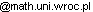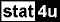### Website of Ryszard Szwarc

 Analiza funkcjonalna 3 Analiza funkcjonalna 2 Analiza funkcjonalna 1 Szeregi Fouriera Odczyty Wielomiany ortogonalne i problem momentów Analiza 3 Analiza 2 Programowanie matematyczne Kombinatoryka ISIM i Analiza I i II Warsztaty w Lubachowie Sport i inne Funkcje analityczne Różne Seminarium magisterskie

### Research

1. Orthogonal polynomials

Ryszard Szwarc
Institute of Mathematics, Wrocław University
pl. Grunwaldzki 2/4, 50-384 Wrocław, Poland
Fax +48 71 375 7429, e-mail Ryszard.SzwarcORCID

### List of publications

1(i). Nonnegative linearization of orthogonal polynomials

1. Strong nonnegative linearization of orthogonal polynomials, "Theory and Applications of Special Functions. A Volume Dedicated to Mizan Rahman" (eds. M. E. H. Ismail and E. Koelink), Developments in Mathematics Vol. 13, Kluwer Acad. Publ. 2005, 461-477.  pdf
2. Orthogonal polynomials and Banach algebras, "Inzell Lectures on Orthogonal Polynomials,", W. zu Castell, F. Filbir, B. Forster (eds.), Advances in the Theory of Special Functions and Orthogonal Polynomials Nova Science Publishers, vol. 2 (2005), 103-139.  pdf.
3. (coauthors F. Filbir, R. Lasser) Hypergroups of compact type, Journal of Computational and Applied Mathematics, 178 (2005), 205-214.  pdf.
4. (coauthors F. Filbir, R. Lasser) Reiter's condition P_1 and approximate identities for hypergroups, Monatshefte für Mathematik 143 (2004), 189-203. pdf.
5. A necessary and sufficient condition for nonnegative linearization of orthogonal polynomials, Constructive Approximation 19 (2003), 565-573.  pdf.
6. (coauthor W. Młotkowski) Nonnegative linearization of polynomials orthogonal with respect to discrete measures, Constructive Approximation 17 (2001), 413-429.  pdf.
8. Nonnegative linearization and q-ultraspherical polynomials, Methods and Applications of Analysis 2 (1995). 399-407.  pdf.
9. Wielomiany ortogonalne i dyskretne zagadnienie brzegowe, (in Polish), Proceedings of the XIII Annual Meeting of Polish Mathematicians, Jachranka 1994, PTM, 181-187.  pdf.
10. Nonnegative linearization of orthogonal polynomials, Colloquium Mathematicum 69 (1995), 309-316.  pdf.
11. Convolution structures and Haar matrices, Journal of Functional Analysis 113 (1993), 19-35.  pdf.
12. Linearization and connection coefficients of orthogonal polynomials, Monatshefte für Mathematik 113 (1992), 319-329.  pdf.
13. Convolution structures associated with orthogonal polynomials, Journal of Mathematical Analysis and Applications 170 (1992), 158-170.  pdf.
14. Orthogonal polynomials and a discrete boundary value problem II, SIAM Journal on Mathematical Analysis 23 (1992), 965-969.  pdf.
15. Orthogonal polynomials and a discrete boundary value problem I, SIAM Journal on Mathematical Analysis 23 (1992), 959-964.  pdf.

1. Connection coefficients of orthogonal polynomials with an application to Legendre polynomials, in Applications of hypergroups and Related Measures Algebras, (ed. A. L. Schwartz et. al.), Seattle 1993, Contemporary Mathematics 183 (1995), 341- 346.  pdf.
2. Connection coefficients of orthogonal polynomials, Canadian Mathematical Bulletin 35 (1992), 548-556.  pdf.

#### 1(iii). Pointwise estimates of orthogonal polynomials

1. (coauthor C. Berg) Self-adjoint operators associated with Hankel moment matricesJournal of Functional Analysis 283 (10)  (2022), pdf
2. (coauthor C. Berg) Closable Hankel operators and  moment problems, Integral Equations and Operator Theory 92(1) (2020), 1-9. pdf
3. (coauthor E. A. van Doorn) On a property of random walk polynomials involving Christoffel functions, Journal of Mathematical Analysis and Applications 477 (1) (2019),  85-103 pdf.
4. (coauthor C. Berg) Inverse of infinite Hankel moment matrices SIGMA 14 (2018), 109. https://www.emis.de/journals/SIGMA/2018/109/
5. Inhomogeneous Jacobi matrices on trees Constructive Approxiamtion 48 (2) (2018), 183–199.. pdf
6. (coauthor C. Berg) Symmetric moment problems and a conjecture of Valent, Matematiceskii Sbornik 208 (2017), 28–53.pdf
7. (coauthor C. Berg) A determinant characterization of moment sequences with finitely many mass-points, Linear and Multilinear Algebra 63 (2015), 1568-1576. pdf
8. (coauthor C. Berg) The order of indeterminate moment problems, Advances in Mathematics 250 (2014),  105-143.  pdf
9. (coauthor C. Berg) The smallest eigenvalue of Hankel matrices, Constructive Approximation 34 (2011),  107-133. pdf
10. coauthor A. Kazun), Jacobi matrices on trees,  Colloquium Mathematicum 118 (2010), 465-497. pdf
11. (coauthor J. Obermaier) Orthogonal polynomials of discrete variable and boundedness of Dirichlet kernel, Constructive Approximation 27 (2008), 1-13.  pdf.
12. (coauthor J. Obermaier) Nonnegative linearization for little q-Laguerre polynomials and Faber basis, Journal of Computational and Applied Mathematics, 199 (2007), 89-94.  pdf.
13. (coauthor J. Obermaier) Polynomial bases for continuous function spaces, in Trends and Applications in Constructive Approximation (Eds.) M.G. de Bruin, D.H.  Mache,  J. Szabados, International Series of Numerical Mathematics Vol. 151, 195-205,  2005 Birkhãuser Verlag Basel.  pdf.
14. Absolute continuity of certain unbounded Jacobi matrices, Advanced Problems in Constructive Approximation, (Eds.) M. D. Buhmann and D. H. Mache, International Series of Numerical Mathematics Vol. 142 (2003), 255-262.  pdf.
15. (coauthors F. Filbir, R. Girgensohn, A. Saxena, A. I. Singh) Simultaneous preservation of orthogonality of polynomials by linear operators arising from dilation of orthogonal polynomial systems, Journal of Computational Analysis and Applications 2 (2000), 177-213.  pdf.
16. (coauthor M.O. Gebuhrer) On symmetry problem for discrete polynomial hypergroups Proceedings of the American Mathematical Society, 127 (1999), 1705-1709.  pdf.
17. A counterexample to subexponential growth of orthogonal polynomials, Constructive Approximation 11 (1995), 381-389.  pdf.
18. Uniform subexponential growth of orthogonal polynomials, Journal of Approximation Theory 81 (1995), 296-302.  pdf.
19. A lower bound for orthogonal polynomials with an application to polynomial hypergroups, Journal of Approximation Theory 81 (1995), 145-150.  pdf.
1(iv). Chain sequences
1. Sharp estimates for Jacobi matrices and chain sequences, II, Journal of Approximation Theory, 125 (2003), 295-302. pdf.
2. Sharp estimates for Jacobi matrices and chain sequences, Journal of Approximation Theory, 118 (2002), 94-105. pdf.
3. Chain sequences, orthogonal polynomials and Jacobi matrices, Journal of Approximation Theory, 92 (1998), 59-73. pdf
4. Chain sequences and compact perturbations of orthogonal polynomials, Mathematische Zeitschrift 217 (1994), 57-71.  pdf.

1(v). Turán determinants
1. Positivity of Turán's determinants for orthogonal polynomials II, Journal of Approximation Theory 270 (2021). pdf
2. (coauthor C. Berg) Bounds on  Turán determinants,  Journal of Approximation Theory 161 (2009), 127–141.  pdf
3. Positivity of Turán's determinants for orthogonal polynomials, in Harmonic Analysis and Hypergroups (K.A. Ross et al., ed.) Delhi 1995, Birkhauser, Boston-Basel-Berlin, 1998, 165-182. pdf.

2. Harmonic analysis of discrete groups
1. (coauthors U. Haagerup, T. Steenstrup)  Schur Multipliers and Spherical Functions on Homogeneous Trees, International Journal of Mathematics, 21 (2010), 1337-1382.  pdf.
2. (coauthor T. Pytlik) Weak type radial convolution operators on free group, Studia Mathematica 186 (2008), 77-86. pdf.
3. Tadek Pytlik in my memories, Colloquium Mathematicum 108 (2007), 1-6.  pdf.
4. (coauthor M. Bożejko) Algebraic length and Poincaré series on reflection groups with applications to representation theory, Asymptotic Combinatorics with Applications to Mathematical Physics, A. M. Vershik (Ed.), Lecture Notes in Mathematics 1815 (2003), 201-221.  pdf.
5. Structure of geodesics in the Cayley graph of infinite Coxeter groups, Colloquium Mathematicum 95 (2003), 79-90.  pdf.
6. Norm estimates of discrete Schrödinger operators, Colloquium Mathematicum 76 (1998), 153-160.  pdf.
7. The ratio and generating function of cogrowth coefficients of finitely generated groups, Studia Mathematica 131 (1998), 89-94.  pdf.
8. (coauthors A. M. Mantero, T. Pytlik and A. Zappa) Equivalence of analytic continuations of the principal series of representations for the free group, Annali di Matematica Pura ed Applicata 165 (1993), 23-28.pdf
9. Groups acting on trees and approximation properties of the Fourier algebra, Journal of Functional Analysis 95 (1991), 320-343.  pdf.
10. Banach algebras associated with the spherical representations of the free group, Pacific Journal of Mathematics 143 (1990), 201-207. pdf.
11. A short proof of the Grigorchuk-Cohen cogrowth theorem, Proceedings of the American Mathematical Society 106 (1989), 663-665. pdf
12. Matrix coefficients of irreducible representations of free products of groups, Studia Mathematica 94 (1989), 179-185. pdf
13. An analytic series of irreducible representations of the free group, Annales de l'Institut Fourier de l'Université Grenoble 38 (1988), 87-110. pdf.
14. (coauthor T. Pytlik) An analytic family of uniformly bounded representations of free groups, Acta Mathematica 157 (1986), 287-309. pdf.
15. Convolution operators of weak type (p,p) which are not of strong type (p,p), Proceedings of the American Mathematical Society 89 (1983), 184-185. pdf
16. Convolution operators of weak type (2,2) which are not of strong type (2,2), Proceedings of the American Mathematical Society 87 (1983), 695-698. pdf

3. Integral inequalities
1. Nonlinear integral inequalities of the Volterra type, Mathematical Proceedings of Cambridge Philosophical Society 111 (1992), 599-608. pdf.
2. Attraction principle for nonlinear integral operators of the Volterra type, Journal of Mathematical Analysis and Applications 170 (1992), 449-456.  pdf.

4. Kaczmarz algorithm
1. Convergence of weighted averages of relaxed projections, Linear and Multilinear Algebra 66 (2018), 1991-2002. pdf
2. (coauthor G. Świderski) Kaczmarz algorithm with relaxation in Hilbert space, Studia Mathematica 216 (2013), 237-243. pdf.
3. Kaczmarz algorithm in Hilbert space and tight frames, Applied and Computational Harmonic Analysis  22 (2007), 382-385.   pdf.
4. (coauthor R. Haller) Kaczmarz algorithm in Hilbert space, Studia Mathematica  169.2 (2005), 123-132. pdf.
1. (coauthors W. zu Castell, F. Filbir) Strictly positive definite functions ind, Journal of Approximation Theory 137 (2005), 277-280. pdf.
6. Other
1. (coauthor W. W. Koczkodaj)  On axiomatization of inconsistency indicators in pairwise comparisons, Fundamenta Informaticae 132 (2014), 485–500. pdf
2. (coauthors R. Khalil, B. Merdas) Erratum to the Khalil  paper "Multipliers of Schatten Classes", Journal of Functional Analysis 63 (1985), 137-138. pdf
3. (coauthor K. Topolski) Limit theorems for stochastic dynamical system arising in Ising model analysis Probability and Mathematical Statistics 28.2 (2008), 257-270. pdf
4. Advanced Problem 6496 American Mathematical Monthly 94.2 (1987), 197-199. pdf

top of page